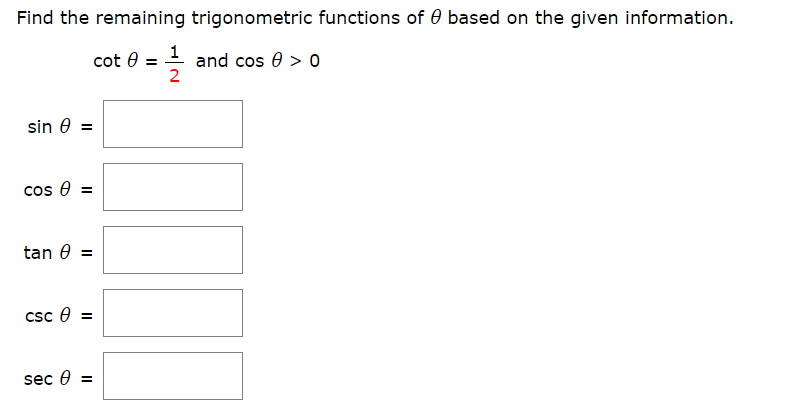# Find the remaining trigonometric functions of 0 based on the given information1cot eand cos 0 > 02=sin e=cos etan ecSc esec e=IlII

Question
4 views

Find the remaining trig functions of (theta) based on the given information

I attached a picture of questionhelp_outlineImage TranscriptioncloseFind the remaining trigonometric functions of 0 based on the given information 1 cot e and cos 0 > 0 2 = sin e = cos e tan e cSc e sec e = Il II fullscreen
check_circle

Step 1

Calculation:

It is given that cot θ = 1/2. That is,

Step 2

Use Pythagorean Theorem and compute the hypotenuse as follows.

(Hypotenuse)2 = (1)2 + (2)2

&nb...

### Want to see the full answer?

See Solution

#### Want to see this answer and more?

Solutions are written by subject experts who are available 24/7. Questions are typically answered within 1 hour.*

See Solution
*Response times may vary by subject and question.
Tagged in

### Trigonometric Ratios